Next: Euler Momentum Theorem Up: Incompressible Inviscid Flow Previous: Streamlines, Stream Tubes, and

# Bernoulli's Theorem

In its most general form, Bernoulli's theorem--which was discovered by Daniel Bernoulli (1700-1783)--states that, in the steady flow of an inviscid fluid, the quantity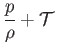(4.2)

is constant along a streamline, whereis the pressure,the density, and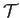the total energy per unit mass.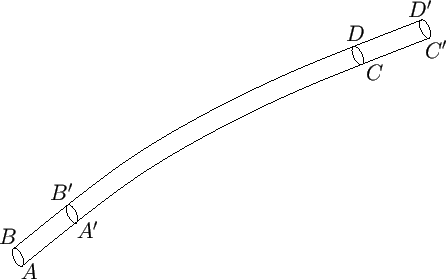The proof is straightforward. Consider the body of fluid bounded by the cross-sectional areas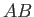and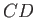of the stream filament pictured in Figure 4.1. Let us denote the values of quantities atandby the suffixesand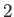, respectively. Thus,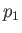,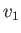,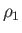,,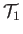are the pressure, flow speed, mass density, cross-sectional area, and total energy per unit mass, respectively, at, et cetera. Suppose that, after a short time interval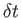, the body of fluid has moved such that it occupies the section of the filament bounded by the cross-sections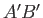and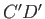, where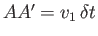and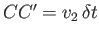. Because the motion is steady, the massof the fluid betweenandis the same as that betweenand, so that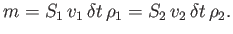(4.3)

Letdenote the total energy of the section of the fluid lying betweenand. Thus, the increase in energy of the fluid body in the time intervalis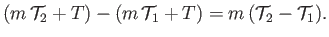(4.4)

In the absence of viscous energy dissipation, this energy increase must equal the net work done on the fluid by the pressures atand, which is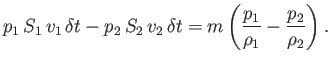(4.5)

Equating expressions (4.4) and (4.5), we find that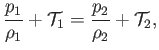(4.6)

which demonstrates that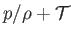has the same value at any two points on a given stream filament, and is therefore constant along the filament. Note that Bernoulli's theorem has only been proved for the case of the steady motion of an inviscid fluid. However, the fluid in question may either be compressible or incompressible.

For the particular case of an incompressible fluid, moving in a conservative force-field, the total energy per unit mass is the sum of the kinetic energy per unit mass,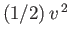, and the potential energy per unit mass,, and Bernoulli's theorem thus becomes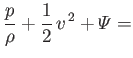constant along a streamline(4.7)

If we focus on a particular streamline, 1 (say), then Bernoulli's theorem states that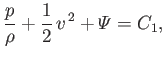(4.8)

where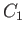is a constant characterizing that streamline. If we consider a second streamline, 2 (say), then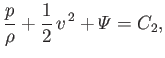(4.9)

where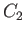is another constant. It is not generally the case that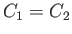. If, however, the fluid motion is irrotational then the constant in Bernoulli's theorem is the same for all streamlines (see Section 4.15), so that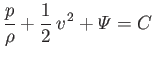(4.10)

throughout the fluid.Next: Euler Momentum Theorem Up: Incompressible Inviscid Flow Previous: Streamlines, Stream Tubes, and
Richard Fitzpatrick 2016-03-31